## Getting My Eye In Around F1 Quali Data – Parallel Coordinate Plots, Sort of…

Looking over the sector times from the qualifying session for tomorrow’s Hungarian Grand Prix, I noticed that Vettel was only fastest in one of the sectors.

Whilst looking for an easy way of shaping an R data frame so that I could plot categorical values sector1, sector2, sector3 on the x-axis, and then a line for each driver showing their time in the sector on the y-axis (I still haven’t worked out how to do that? Any hints? Add them to the comments please…;-), I came across a variant of the parallel coordinate plot hidden away in the lattice package:What this plot does is for each row (i.e. each driver) take values from separate columns (i.e. times from each sector), normalise them, and then plot lines between the normalised value, one “axis” per column; each row defines a separate category.

The normalisation obviously hides the magnitude of the differences between the time deltas in each sector (the min-max range might be hundredths in one sector, tenths in another), but this plot does show us that there are different groupings of cars – there is clear(?!) white space in the diagram:Whilst the parallel co-ordinate plot helps identify groupings of cars, and shows where they may have similar performance, it isn’t so good at helping us get an idea of which sector had most impact on the final lap time. For this, I think we need to have a single axis in seconds showing the delta from the fastest time in the sector. That is, we should have a parallel plot where the parallel axes have the same scale, but in terms of sector time, a floating origin (so e.g. the origin for one sector might be 28.6s and for another, 22.4s). For convenience, I’d also like to see the deltas shown on the y-axis, and the categorical ranges arranged on the x-axis (in contrast to the diagrams above, where the different ranges appear along the y-axis).

PS I also wonder to what extent we can identify signatures for the different teams? Eg the fifth and sixth slowest cars in sector 1 have the same signature across all three sectors and come from the same team; and the third and fourth slowest cars in sector 2 have a very similar signature (and again, represent the same team).

Where else might we look for signatures? In the speed traps maybe? Here’s what the parallel plot for the speed traps looks like: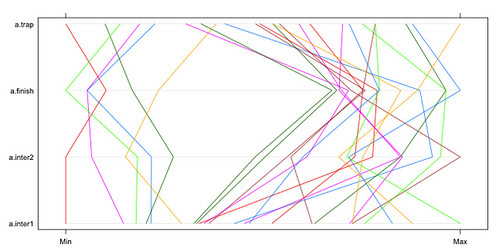(In this case, max is better = faster speed.)

To combine the views (timings and speed), we might use a formulation of the flavour:

parallel(~data.frame(a\$sector1,a\$sector2,a\$sector3, -a\$inter1,-a\$inter2,-a\$finish,-a\$trap))This is a bit too cluttered to pull much out of though? I wonder if changing the order of parallel axes might help, e.g. by trying to come up with an order than minimises the number of crossed lines?

And if we colour lines by team, can we see any characteristics?Using a dashed, rather than solid, line makes the chart a little easier to read (more white space). Using a thinking line also helps bring out the colours.

parallel(~data.frame(a\$sector1,-a\$inter1,-a\$inter2,a\$sector2,a\$sector3, -a\$finish,-a\$trap),col=a\$team,lty=2,lwd=2)

Here’s another ordering of the axes:Here are the sector times ordered by team (min is better):Here are the speeds by team (max is better):Again, we can reorder this to try to make it easier(?!) to pull out team signatures:(I wonder – would it make sense to try to order these based on similarity eg derived from a circuit guide?)

Hmmm… I need to ponder this…

## Merging Two Different Datasets Containing a Common Column With R and R-Studio

Another way for the database challenged (such as myself!) for merging two datasets that share at least one common column…

This recipe using the cross-platform stats analysis package, R. I use R via the R-Studio client, which provides an IDE wrapper around the R environment.

So for example, here’s how to merge a couple of files sharing elements in a common column…

First, load in your two data files – for example, I’m going to load in separate files that contain qualifying and race stats from the last Grand Prix: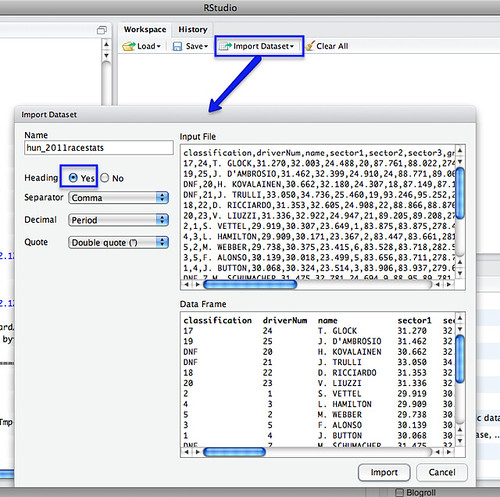We can merge the datasets using a command of the form:

m=merge(hun_2011racestats,hun_2011qualistats,by="driverNum")

The by parameter identifies which column we want to merge the tables around. (If the two datasets have different column names, you need to set by.x= and by.y= to specify the column from each dataset that is the focus for merging).

So for example, in the simple case where we are merging around two columns of the same name in different tables:

After the merge, column names for columns from the first table have the .x suffix added, and from the second, .y.

We can then export the combined dataset as a CSV file:

write.csv(m, file = "demoMerge.csv")[If you want to extract a subset of the columns, specify the required columns in an R command of the form: m2=m[c(“driverNum”,”name.x”,”ultimate.x”,”ultimate.y”)] See also: R: subset]

Simples;-)

PS in the above example, the merge table only contains merged rows. If there are elements in the common column of one table, but not the other, that partial data will not be included in the merged table. To include all rows, set all=TRUE. To include all rows from the first table, but not unmatched rows from the second, set all.x=TRUE; (the cells from columns in the unmatched row of the second table will be set to NA). (all.y=TRUE is also legitimate). From the R merge documentation:

In SQL database terminology, the default value of all = FALSE [the default] gives a natural join, a special case of an inner join. Specifying all.x = TRUE gives a left (outer) join, all.y = TRUE a right (outer) join, and both (all=TRUE a (full) outer join. DBMSes do not match NULL records, equivalent to incomparables = NA in R.

For other ways of combining data from two different data sets, see:
Merging Datasets with Common Columns in Google Refine
A Further Look at the Orange Data Playground – Filters and File Merging
Merging CSV data files with Google Fusion Tables

If you know of any other simple ways of joining data files about a common column, please reveal all in the comments:-)

## Data Driven Story Discovery: Working Up a Multi-Layered Chart

How many different dimensions (or “columns” in a dataset where each row represents a different sample and each column a different measurement taken as part of that sample) can you plot on a chart?

Two are obvious: X and Y values, which are ideal for representing continuous numerical variables. If you’re plotting points, as in a scatterplot, the size and the colour of the point allow you to represent two further dimensions. Using different symbols to plot the points gives you another dimension. So we’re up to five, at least.

Whilst I was playing with ggplot over the weekend, I fell into this view over F1 timing data:It shows the range of positions held by each car over the course of the race (cars are identified by car number on the x-axis, 1 to 25 (there is no 13), and range of positions on the y-axis. The colour, uninterestingly, relates to car number.

If you follow any of the F1 blogs, you’ll quite often see references to “driver of the day” discussions (e.g. Who Was Your Driver of the Day). Which got me wondering…could a variant of the above chart provide a summary of the race as a whole that would, at a glance, suggest which drivers had “interesting” races?

For example, if a driver took on a wide range of race positions during the race, might this mean they had an exciting time of it? If every car retained the same couple of positions throughout the race, was it a procession?

Having generated the base chart using the ggplot web interface, I grabbed the code used to generate it and took it into RStudio.

What was lacking in the original view was an explicit statement about the position of each car at the end of the race. But we can add this using a point, if we know the final race position*:ggplot() + geom_step(aes(x=h\$car, y=h\$pos, group=h\$car)) + scale_x_discrete(limits = c('VET','WEB','HAM','BUT','ALO','MAS','SCH','ROS','HEI','PET','BAR','MAL','','SUT','RES','KOB','PER','BUE','ALG','KOV','TRU','RIC','LIU','GLO','AMB')) + xlab(NULL) + opts(axis.text.x=theme_text(angle=-90, hjust=0)) + geom_point(aes(x=k\$driverNum, y=k\$classification))

Let’s add it using a different coloured mark:+geom_point(aes(x=k\$driverNum, y=k\$grid,col='red'))

(Note that in the above example, the points are layered in the order they appear in the command line, with lower layers first. So if a car finishes (black dot) in the position it started (red dot), we will only see the red dot. If we make the lower layer dot slightly larger, we would then get a target effect in this case.)

The chart as we now have it shows where a driver started, where they finished and how many different race positions they found themselves in. So for example, we see BUE rapidly improved on his grid position at the start of the race and made progress through the race, but SUT went backwards from the off, as did PER.

Hmm… lots of race action seems to have happened during the first lap, but we’re not getting much sense of it… Maybe we need to add in the position of the car at the end of the first lap too. Unfortunately, the data I was using did not contain the actual grid/starting position (it just contains the positions at the end of each lap), but we can pull this data in from elsewhere… Using another dot to represent this piece of data might get confusing, so let’s use a line (set the symbol type using pch=3):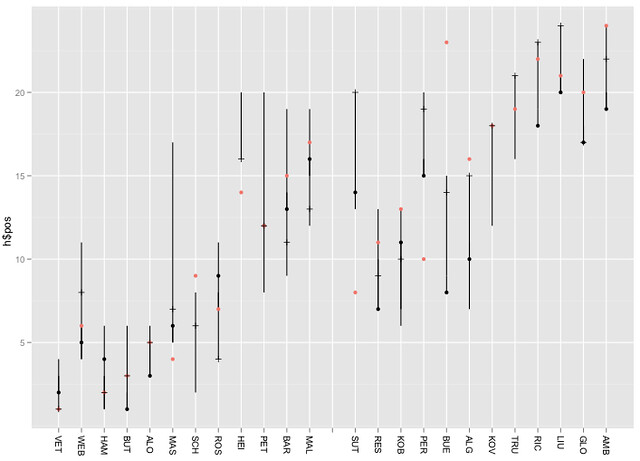+geom_point(aes(x=l\$car, y=l\$pos, pch=3))

Now we have a chart that shows the range of positions occupied by each car, their grid position, final race position and position at the end of the first lap Using different symbol types and colours we can distinguish between them. (For a presentation graphic, we also need a legend…) By using different sized symbols and layers, we could also display multiple dimensions at the same x, y co-ordinate.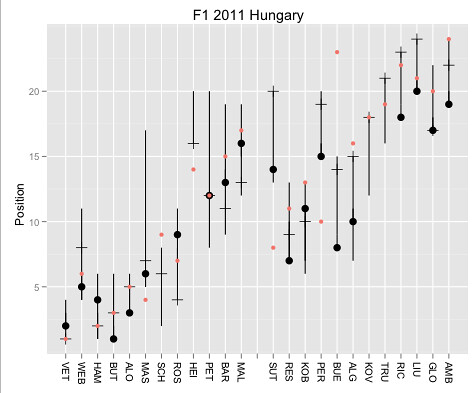library("ggplot2")
h=hun_2011proximity
//in racestats, convert DNF etc to NA
k=hun_2011racestatsx
l=subset(h,lap==1)
ggplot() + geom_step(aes(x=h\$car, y=h\$pos, group=h\$car)) + scale_x_discrete(limits =c('VET','WEB','HAM','BUT','ALO','MAS','SCH','ROS','HEI','PET','BAR','MAL','','SUT','RES','KOB','PER','BUE','ALG','KOV','TRU','RIC','LIU','GLO','AMB')) + xlab(NULL) + opts(title="F1 2011 Hungary", axis.text.x=theme_text(angle=-90, hjust=0)) + geom_point(aes(x=l\$car, y=l\$pos, pch=3, size=2)) + geom_point(aes(x=k\$driverNum, y=k\$classification,size=2), label='Final') + geom_point(aes(x=k\$driverNum, y=k\$grid, col='red')) + ylab("Position")

A title can be added to the post using:

I still haven’t worked out how to do a caption that will display:
– [black dot] “Final Race Position”
– [red dot] “Grid Position”
– [tick mark] “End of lap 1”

Any ideas?

The y-axis gridline on the half is also misleading. To add gridlines for each position, add in +scale_y_discrete(breaks=1:24) or to label them as well +scale_y_discrete(breaks=1:24,limits=1:24)So what do we learn from this? Layers can be handy and allow us to construct charts with different overlaid data sets. The order of the layers can make things easier or harder to read. Different symbol types work differently well with each other when overlaid. The same symbol shape in different sizes and appropriate layer ordering allows you to overlay points and still see them data. Symbol styles give the chart a grammar (I used circles for the start and end of the race positions, for example, and a line for the first lap position).

Lots of little things, in other words. But lots of little things that can allow us to add more to a chart and still keep it legible (arguably! I don’t claim to be a graphic designer, I’m just trying to find ways of communicating different dimensions within a data set).

* it strikes me that actually we could plot the final race position under the final classification. (On occasion, the race stewards penalise drivers so the classified result is different to the positions the cars ended the race in. Maybe we should make that eventuality evident?)

PS as @sidepodcast pointed out, I really should remove non-existent driver 13. I guess this means converting x-axis to a categorical one?

## The Visual Difference – R and Anscombe’s Quartet

I spent a chunk of today trying to get my thoughts in order for a keynote presentation at next week’s The Difference that Makes a Difference conference. The theme of my talk will be on how visualisations can be used to discover structure and pattern in data, and as in many or my other recent talks I found the idea of Anscombe’s quartet once again providing a quick way in to the idea that sometimes the visual dimension can reveal a story that simple numerical analysis appears to deny.

For those of you who haven’t come across Anscombe’s quartet yet, it’s a set of four simple 2 dimensional data sets (each 11 rows long) that have similar statistical properties, but different stories to tell…

Quite by chance, I also happened upon a short exercise based on using R to calculate some statistical properties of the quartet (More useless statistics), so I thought I’d try and flail around in my unprincipled hack-it-and-see approach to learning R to see if I could do something similar with rather simpler primitives than described in that blog post.

(If you’re new to R and want to play along, I recommend RStudio…)

Here’s the original data set – you can see it in R simply by typing anscombe:

```   x1 x2 x3 x4    y1   y2    y3    y4
1  10 10 10  8  8.04 9.14  7.46  6.58
2   8  8  8  8  6.95 8.14  6.77  5.76
3  13 13 13  8  7.58 8.74 12.74  7.71
4   9  9  9  8  8.81 8.77  7.11  8.84
5  11 11 11  8  8.33 9.26  7.81  8.47
6  14 14 14  8  9.96 8.10  8.84  7.04
7   6  6  6  8  7.24 6.13  6.08  5.25
8   4  4  4 19  4.26 3.10  5.39 12.50
9  12 12 12  8 10.84 9.13  8.15  5.56
10  7  7  7  8  4.82 7.26  6.42  7.91
11  5  5  5  8  5.68 4.74  5.73  6.89```

We can construct a simple data frame containing just the values of x1 and y1 with a construction of the form: data.frame(x=c(anscombe\$x1),y=c(anscombe\$y1)) (where we identify the columns explicitly by column name) or alternatively data.frame(x=c(anscombe),y=c(anscombe)) (where we refer to them by column index number).

x y
1 10 8.04
2 8 6.95
3 13 7.58
4 9 8.81
5 11 8.33
6 14 9.96
7 6 7.24
8 4 4.26
9 12 10.84
10 7 4.82
11 5 5.68

A tidier way of writing this is as follows:
with(anscombe,data.frame(x1Val=c(x1),y1Val=c(y1)))

In order to call on, or refer to, the data frame, we assign it to a variable: g1data=with(anscombe,data.frame(xVal=c(x1),yVal=c(y1)))

We can then inspect the mean and sd values: mean(g1data\$xVal), or sd(g1data\$yVal)

> mean(g1data\$xVal)
 9
> sd(g1data\$xVal)
 3.316625
>

To plot the data, we can simply issue a plot command: plot(g1data)It would be possible to create similar datasets for each of the separate groups of data, but R has all sorts of tricks for working with data (apparently…?!;-) There are probably much better ways of getting hold of the statistics in a more direct way, but here’s the approach I took. Firstly, we need to reshape the data a little. I cribbed the “useless stats” approach for most of this. The aim is to produce a data set with 44 rows, and 3 columns: x, y and a column that identifies the group of results (let’s call them myX, myY and myGroup for clarity). The myGroup values will range from 1 to 4, identifying each of the four datasets in turn (so the first 11 rows will be for x1, y1 and will have myGroup value 1; then the values for x2, y2 and myGroup equal to 2, and so on). That is, we want a dataset that starts:

```1 10 9.14 1
2 8 8.14 1```

and ends:

```43 8 7.91 4
44 8 6.89 4```

To begin with, we need some helper routines:

– how many rows are there in the data set? nrow(anscombe)
– how do we create a set of values to label the rows by group number (i.e. how do we generate a set of 11 1’s, then 11 2’s, 11 3’s and 11 4’s)? Here’s how: gl(4, nrow(anscombe)) [typing ?gl in R should bring up the appropriate help page;-) What we do is construct a list of 4 values, with each value repeating nrow(anscombe) times]
– to add in a myGroup column to a dataframe containing x1 and y1 columns, set with just values 1, we simply insert an additional column definition: data.frame(xVal=c(anscombe\$x1), yVal=c(anscombe\$y1), mygroup=gl(1,nrow(anscombe)))
– to generate a data frame containing three columns and the data for group 1, followed by group 2, we would use a construction of the form: data.frame(xVal=c(anscombe\$x1,anscombe\$x2), yVal=c(anscombe\$y1,anscombe\$y2), mygroup=gl(2,nrow(anscombe))). That is, populate the xVal column with rows from x1 in the anscombe dataset, then the rows from column x2; populate yVal with values from y1 then y2; and populate myGroup with 11 1’s followed by 11 2’s.
– a more compact way of constructing the data frame is to specify that we want to concatenate (c()) values from several columns from the same dataset: with(anscombe,data.frame(xVal=c(x1,x2,x3,x4), yVal=c(y1,y2,y3,y4), mygroup=gl(4,nrow(anscombe))))
– to be able to reference this dataset, we need to assign it to a variable: mydata=with(anscombe,data.frame(xVal=c(x1,x2,x3,x4), yVal=c(y1,y2,y3,y4), mygroup=gl(4,nrow(anscombe))))

This final command will give us a data frame with the 3 columns, as required, containing values from group 1, then group 2, then groups 3 and 4, all labelled appropriately.

To find the means for each column by group, we can use the aggregate command: aggregate(.~mygroup,data=mydata,mean)

(I think you read that as follows:”aggregate the current data (.) by each of the groups in (~) mygroup using the mydata dataset, reporting on the groupwise application of the function: mean)

To find the SD values: aggregate(.~mygroup,data=mydata,sd)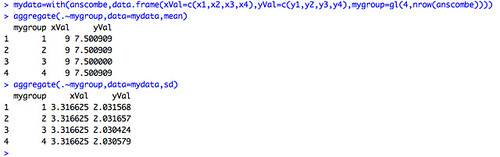Cribbing an approach I discovered from the hosted version of the ggplot R graphics library, here’s a way of plotting the data for each of the four groups from within the single aggregate dataset. (If you are new to R, you will need to download and install the ggplot2 package; in RStudio, from the Packages menu, select Install Packages and enter ggplot2 to download and install the package. To load the package into your current R session, tick the box next to the installed package name or enter the command library("ggplot2").)

The single command to plot xy scatterplots for each of the four groups in the combined 3 column dataset is as follows:

ggplot(mydata,aes(x=xVal,y=yVal,group=mygroup))+geom_point()+facet_wrap(~mygroup)

And here’s the result (remember, the statistical properties were the same…)To recap the R commands:

mydata=with(anscombe,data.frame(xVal=c(x1,x2,x3,x4), yVal=c(y1,y2,y3,y4), group=gl(4,nrow(anscombe))))
aggregate(.~mygroup,data=mydata,mean)
aggregate(.~mygroup,data=mydata,sd)
library(ggplot2)
ggplot(mydata,aes(x=xVal, y=yVal)) + geom_point() + facet_wrap(~mygroup)

PS this looks exciting from an educational and opendata perspective, though I haven’t had a chance to play with it: OpenCPU: a server where you can upload and run R functions. (The other hosted R solutions I was aware of – R-Node – doesn’t seem to be working any more? online R-Server [broken?]. For completeness, here’s the link to the hosted ggplot IDE referred to in the post. And finally – if you need to crucnh big files, CloudNumbers may be appropriate (disclaimer: I haven’t tried it))

PPS And here’s something else for the data junkies – an easy way of getting data into R from Datamarket.com: How to access 100M time series in R in under 60 seconds.

I couldn’t contain myself (other more pressing things to do, but…), so I just took a quick time out and a coffee to put together a quick and dirty R function that will let me run queries over Google spreadsheet data sources and essentially treat them as database tables (e.g. Using Google Spreadsheets as a Database with the Google Visualisation API Query Language).

Here’s the original function I used:

```library(RCurl)
```

However, with a move to https, this function kept breaking. The one I currently use is:

```library(RCurl)
gsqAPI = function(key,query,gid=0){
tmp=getURL( paste( sep="",'https://spreadsheets.google.com/tq?', 'tqx=out:csv','&tq=', curlEscape(query), '&key=', key, '&gid=', gid), ssl.verifypeer = FALSE )
return( read.csv( textConnection( tmp ) ) )
}
```

It requires the spreadsheet key value and a query; you can optionally provide a sheet number within the spreadsheet if the sheet you want to query is not the first one.

We can call the function as follows:

gsqAPI('tPfI0kerLllVLcQw7-P1FcQ','select * limit 3')

In that example, and by default, we run the query against the first sheet in the spreadsheet.

Alternatively, we can make a call like this, and run a query against sheet 3, for example:
tmpData=gsqAPI('0AmbQbL4Lrd61dDBfNEFqX1BGVDk0Mm1MNXFRUnBLNXc','select A,C where <= 10',3)
tmpDataThe real question is, of course, could it be useful.. (or even OUseful?!)?

Here’s another example: a way of querying the Guardian Datastore list of spreadsheets:

gsqAPI('0AonYZs4MzlZbdFdJWGRKYnhvWlB4S25OVmZhN0Y3WHc','select * where A contains "crime" and B contains "href" order by C desc limit 10')

What that call does is run a query against the Guardian Datastore spreadsheet that lists all the other Guardian Datastore spreadsheets, and pulls out references to spreadsheets relating to “crime”.

The returned data is a bit messy and requires parsing to be properly useful.. but I haven’t started looking at string manipulation in R yet…(So my question is: given a dataframe with a column containing things like <a href=”http://example.com/whatever”>Some Page</a>, how would I extract columns containing http://example.com/whatever or Some Page fields?)

[UPDATE: as well as indexing a sheet by sheet number, you can index it by sheet name, but you’ll probably need to tweak the function to look end with '&gid=', curlEscape(gid) so that things like spaces in the sheet name get handled properly I’m not sure about this now.. calling sheet by name works when accessing the “normal” Google spreadsheets application, but I’m not sure it does for the chart query language call??? ]

[If you haven’t yet discovered R, it’s an environment that was developed for doing stats… I use the RStudio environment to play with it. The more I use it (and I’ve only just started exploring what it can do), the more I think it provides a very powerful environment for working with data in quite a tangible way, not least for reshaping it and visualising it, let alone doing stats with in. (In fact, don’t use the stats bit if you don’t want to; it provides more than enough data mechanic tools to be going on with;-)]

PS By the by, I’m syndicating my Rstats tagged posts through the R-Bloggers site. If you’re at all interested in seeing what’s possible with R, I recommend you subscribe to R-Bloggers, or at least have a quick skim through some of the posts on there…

PPS The RSpatialTips post Accessing Google Spreadsheets from R has a couple of really handy tips for tidying up data pulled in from Google Spreadsheets; assuming the spreadsheetdata has been loaded into ssdata: a) tidy up column names using colnames(ssdata) <- c("my.Col.Name1","my.Col.Name2",...,"my.Col.NameN"); b) If a column returns numbers as non-numeric data (eg as a string "1,000") in cols 3 to 5, convert it to a numeric using something like: for (i in 3:5) ssdata[,i] <- as.numeric(gsub(",","",ssdata[,i])) [The last column can be identifed as ncol(ssdata) You can do a more aggessive conversion to numbers (assuming no decimal points) using gsub("[^0-9]","",ssdata[,i])]

PPPS via Revolutions blog, how to read the https file into R (unchecked):

```require(RCurl)
myCsv = getURL(httpsCSVurl)

In Using Google Spreadsheets as a Database Source for R, I described a simple Google function for pulling data into R from a Google Visualization/Chart tools API query language query applied to a Google spreadsheet, given the spreadsheet key and worksheet ID. But how do you get a list of sheets in spreadsheet, without opening up the spreadsheet and finding the sheet names or IDs directly? [Update: I’m not sure the query language API call lets you reference a sheet by name…]

To look up the sheets associated with a spreadsheet identified by its key value KEY, construct a URL of the form:

This should give you an XML output. To get the output as a JSON feed, append ?alt=json to the end of the URL.

Having constructed the URL for sheets listing for a spreadsheet with a given key identifier, we can pull in and parse either the XML version, or the JSON version, into R and identify all the different sheets contained within the spreadsheet document as a whole.

First, the JSON version. I use the RJSONIO library to handle the feed:

library(RJSONIO)
sskey='0AmbQbL4Lrd61dDBfNEFqX1BGVDk0Mm1MNXFRUnBLNXc'
sheets=c()
as.data.frame(sheets)

Using a variant of the function described in the previous post, we can look up the data contained in a sheet by the sheet ID (I’m not sure you can look it up by name….?) – I’m not convinced that the row number is a reliable indicator of sheet ID, especially if you’ve deleted or reordered sheets. It may be that you do actually need to go to the spreadsheet to look up the sheet number for the gid, which actually defeats a large part of the purpose behind this hack?:-(

library(RCurl)
gsqAPI = function( key, query,gid=0){ return( read.csv( paste( sep="", 'http://spreadsheets.google.com/tq?', 'tqx=out:csv', '&tq=', curlEscape(query), '&key=', key, '&gid=', curlEscape(gid) ) ) ) }
gsqAPI(sskey,"select * limit 10", 9)The second approach is to pull on the XML version of the sheet data feed. (This PDF tutorial got me a certain way along the road: Extracting Data from XML, but then I got confused about what to do next (I still don’t have a good feel for identifying or wrangling with R data structures, though at least I now know how to use the class() function to find out what R things the type of any given item is;-) and had to call on the lazy web to work out how to do this in the end!)

library(XML)